# S12E2 - Rate Laws in Chemistry

There are Two Types of Rate Laws in Chemistry.

• Differential Rate Law (or "Rate Law"), and...
• Integrated Rate Law

We'll take a look at each of these in great detail.  Both today in this video blog post, and in the blog posts that follow...

==========

## Rate Laws

To avoid complications due to reversible reactions, we will only study reactions soon after the forward reaction begins.

So... negligible reverse reaction.

----------

ex:  Consider the reaction we originally saw in my last post on this topic, Chemical Kinetics and Reaction Rates (Table 12-1, Figure 12-1):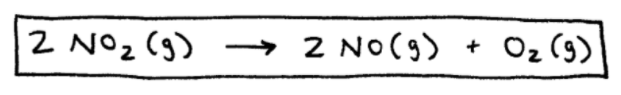Because we're only looking at reactions right after t = 0, the reaction rate only depends on the concentration of our reactant NO2.

Why?

Because there are essentially no products immediately after time = 0.

Okay, here's the rate law for the decomposition of NO2 ...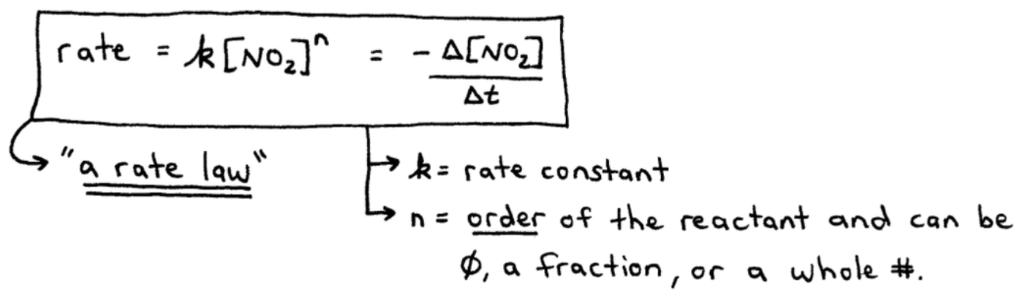➞ k  =  rate constant and can have a variety of different units.

➞ n  =  the order of the reactant, and its value is often 0, 1, or 2.

==========

## Types of Rate Laws

As mentioned earlier, there are two types of rate laws in Chemistry.

Each of them are discussed below.

==========

## Differential Rate Law

Differential Rate Law  =  expresses how the reaction rate depends on the concentration of reactant(s).

➞ Often simply called "the rate law" because it's the most common type of rate law.

See the example here: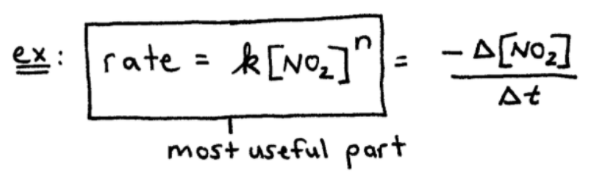==========

## Integrated Rate Law

➞ Integrated Raw Law  =  expresses how the reactant(s) concentration depends on time.

We'll see later that if you know the form of the differential rate law, you automatically know the form of the integrated rate law. (and vice-versa).

----------

So, to summarize:

The differential rate law tells you how the rate of a reaction depends on the concentration of reactant(s).

The integrated rate law tells you how the concentration of reactant(s) depends on time.

----------

➞  QUESTION:  Why is it important to know the rate law of a reaction?

➞  ANSWER:  Because using the known rate law, a chemist can work backwards to learn the individual steps and mechanism by which a reaction occurs.

==========

## How to Determine the Rate Law

We want to determine the form of the rate law because it's the first step in understanding how a chemical reaction occurs.

Take a look at the rate law example below...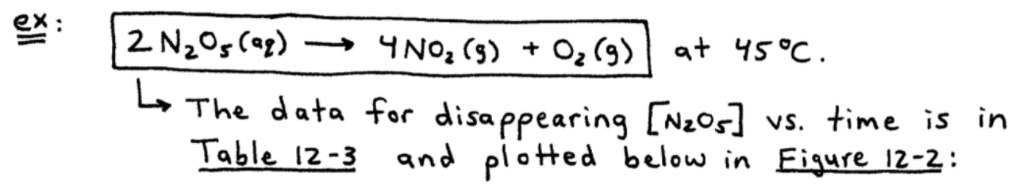The data points in Table 12-3 show that the concentration of N2O5 decreases (y-axis) with increasing time (x-axis).

The results are displayed in Figure 12-2 below...In Figure 12-2 above, you'll see we have drawn 2 tangent lines at 2 data points on the curve (hand-drawn X's).

Let's take a look at those two data points:

----------

➞ at [N2O5]  =  0.90M, the reaction rate is:
5.4 x 10-4 mol/L.s

➞ at [N2O5]  =  0.45M, the reaction rate is:
2.7 x 10-4 mol/L.s

----------

As the concentration is decreased by a factor of 2 (cut in half), so is the reaction rate.

So we have n = 1.

Since the value of "n" is 1, the reaction is first order in N2O5.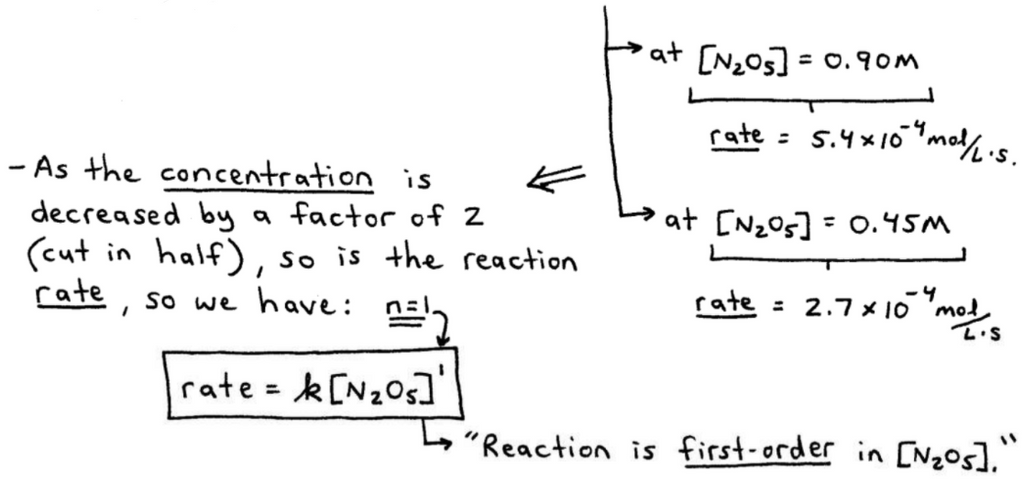==========

Not enough kinetics details for you?

That's because you haven't seen the next video in the sequence...

So stick around for more of "SECTION 12 - Chemical Kinetics."

We'll discuss how to determine the form of the rate law experimentally using the Method of Initial Rates.